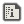# Array insert and remove functions12Me21Created:
These will work on any type of 1D array.

# Insert

```DEF INSERT ARRAY[],POS%,VALUE
UNSHIFT ARRAY,VALUE 'This is just to make the array longer. I use VALUE since it will be the same variable type as the array.
COPY ARRAY,ARRAY,1,POS% 'Moves all the elements before POS% 1 space to the left.
END```

# Remove

```DEF REMOVE ARRAY[],POS%
COPY ARRAY,POS%,ARRAY,POS%+1,LEN(ARRAY)-(POS%+1) 'move all elements after POS% 1 space left.
IF POP(ARRAY) THEN ENDIF'remove the last element.
END```I have found this so useful, and have come back to this resource numerous times. It is much more efficient than looping through every element. I'm putting this minified code here for future reference, also because INSERT is 0.0006 milliseconds faster and REMOVE is 0.0012 milliseconds faster, that's right, faster than 12Me21```'INSERT AN ITEM INTO AN ARRAY
DEF INSERT A[],I%,V
UNSHIFT A,V COPY A,A,1,I% A[I%]=V
END

'REMOVE AN ELEMENT FROM AN ARRAY
DEF REMOVE A[],I%
COPY A,I%,A,I%+1,LEN(A)-I%-1 I%=POP(A)
END```Replying to:Simeon
I have found this so useful, and have come back to this resource numerous times. It is much more efficient than looping through every element. I'm putting this minified code here for future reference, also because INSERT is 0.0006 milliseconds faster and REMOVE is 0.0012 milliseconds faster, that's right, faster than 12Me21```'INSERT AN ITEM INTO AN ARRAY
DEF INSERT A[],I%,V
UNSHIFT A,V COPY A,A,1,I% A[I%]=V
END

'REMOVE AN ELEMENT FROM AN ARRAY
DEF REMOVE A[],I%
COPY A,I%,A,I%+1,LEN(A)-I%-1 I%=POP(A)
END```
The remove function won't work with string array, but yes, that version is faster for integer or float arrays.Replying to:Simeon
I have found this so useful, and have come back to this resource numerous times. It is much more efficient than looping through every element. I'm putting this minified code here for future reference, also because INSERT is 0.0006 milliseconds faster and REMOVE is 0.0012 milliseconds faster, that's right, faster than 12Me21```'INSERT AN ITEM INTO AN ARRAY
DEF INSERT A[],I%,V
UNSHIFT A,V COPY A,A,1,I% A[I%]=V
END

'REMOVE AN ELEMENT FROM AN ARRAY
DEF REMOVE A[],I%
COPY A,I%,A,I%+1,LEN(A)-I%-1 I%=POP(A)
END```
Oh yeah... you're rightSo I figured out, if you use these functions way too often, on huge arrays, they actually work in something like O(n/9000) time complexity, and slow down more and more But if you're only using INSERT and REMOVE on 0 or the end of the array for most of the time, this will make the function work in O(1) time complexity for those cases (which can be an absolutely huge performance increase depending on what you're doing).
```'INSERT AN ITEM INTO AN ARRAY
DEF INSERT A[],I%,V
IF !I% THEN
UNSHIFT A,V
ELSEIF LEN(A)==I% THEN
PUSH A,V
ELSE
UNSHIFT A,V COPY A,A,1,I% A[I%]=V
ENDIF
END

'REMOVE AN ELEMENT FROM AN ARRAY
DEF REMOVE A[],I%
IF !I% THEN
IF SHIFT(A) THEN ENDIF
ELSEIF LEN(A)==I% THEN
IF POP(A) THEN ENDIF
ELSE
COPY A,I%,A,I%+1,LEN(A)-I%-1
IF POP(A) THEN ENDIF
ENDIF
END```
It's a simple, self explanatory solution, but I just thought I'd point this out, since without it, my 3D engine takes about 12 extra seconds to start up, after lots of debugging this was the slowest part of my app, and this fix makes the startup time almost instant. Hope this helps someone!